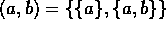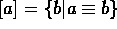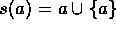Next: Construction of N Up: What are numbers? Previous: Introduction

## Construction of the Number System

Formally (following the mainstream in math) the numbers are constructed from scratch out of the axioms of Zermelo Fraenkel set theory (a.k.a. ZF set theory) [Enderton77, Henle86, Hrbacek84]. The only things that can be derived from the axioms are sets with the empty set at the bottom of the hierarchy. This will mean that any number is a set (it is the only thing you can derive from the axioms). It doesn't mean that you always have to use set notation when you use numbers: just introduce the numerals as an abbreviation of the formal counterparts.

The construction starts with N and algebraically speaking, N with its operations and order is quite a weak structure. In the following constructions the structures will be strengthen one step at the time: Z will be an integral domain, Q will be a field, for the field R the order will be made complete, and field C will be made algebraically complete.

Before we start, first some notational stuff:

• a pair,
• an equivalence class,
• the successor of a is.
Although the previous notations and the constructions that follow are the de facto standard ones, there are different definitions possible.

Alex Lopez-Ortiz
Mon Feb 23 16:26:48 EST 1998